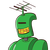# Suppose that a person deposits \$10,000 in a savings account at a bank yielding 11% per year with interest compounded a

Suppose that a person deposits \$10,000 in a savings account at a bank yielding 11% per year

with interest compounded annually. How much will be in the account after 30 years?​

### 1 thought on “Suppose that a person deposits \$10,000 in a savings account at a bank yielding 11% per year <br /><br />with interest compounded a”

1.Step-by-step explanation:

For this problem, use the Annual Compound Interest formula.

A = P(1 + r/n)nt

Here P is the principal amount, P = \$10,000, r is the rate in decimals, r = 0.11, n is the number of compounding periods per unit t, t is time, A is the accrued amount.

Now can you calculate it?

Hint: since you are compounding annually, n = 1, and t = 10 (for 10 years), t = 20 (for 20 years), etc.

Let me know if you need further help.